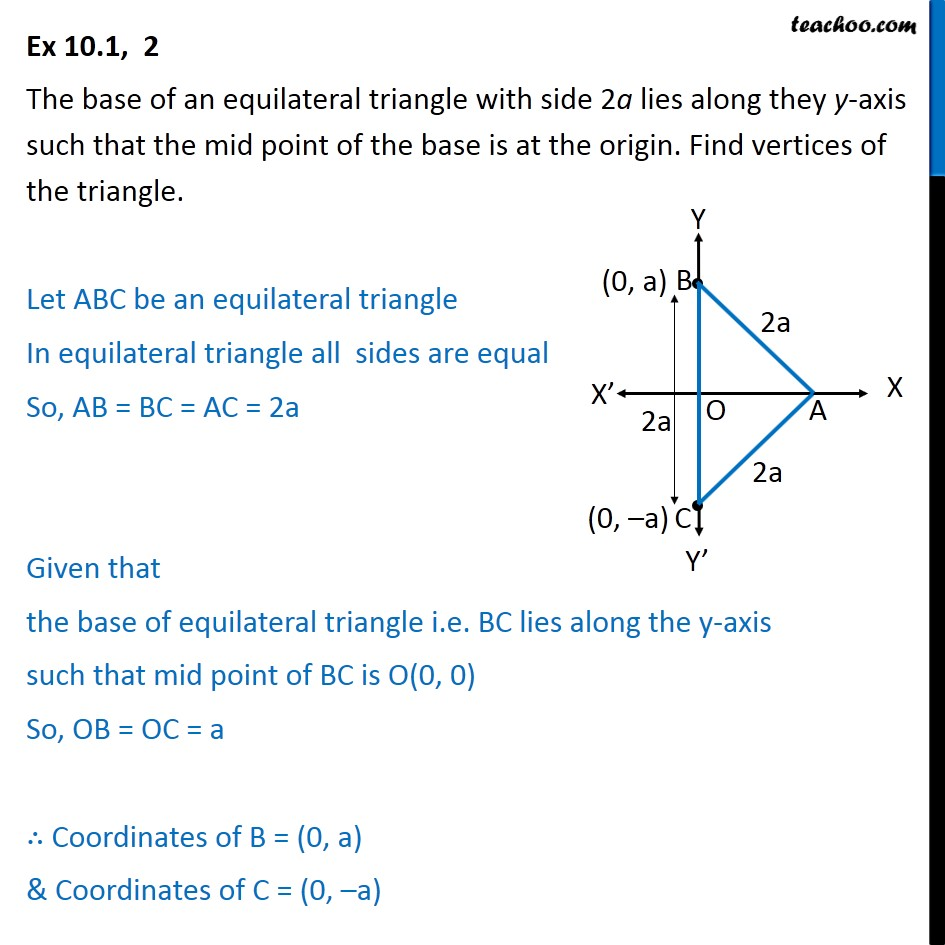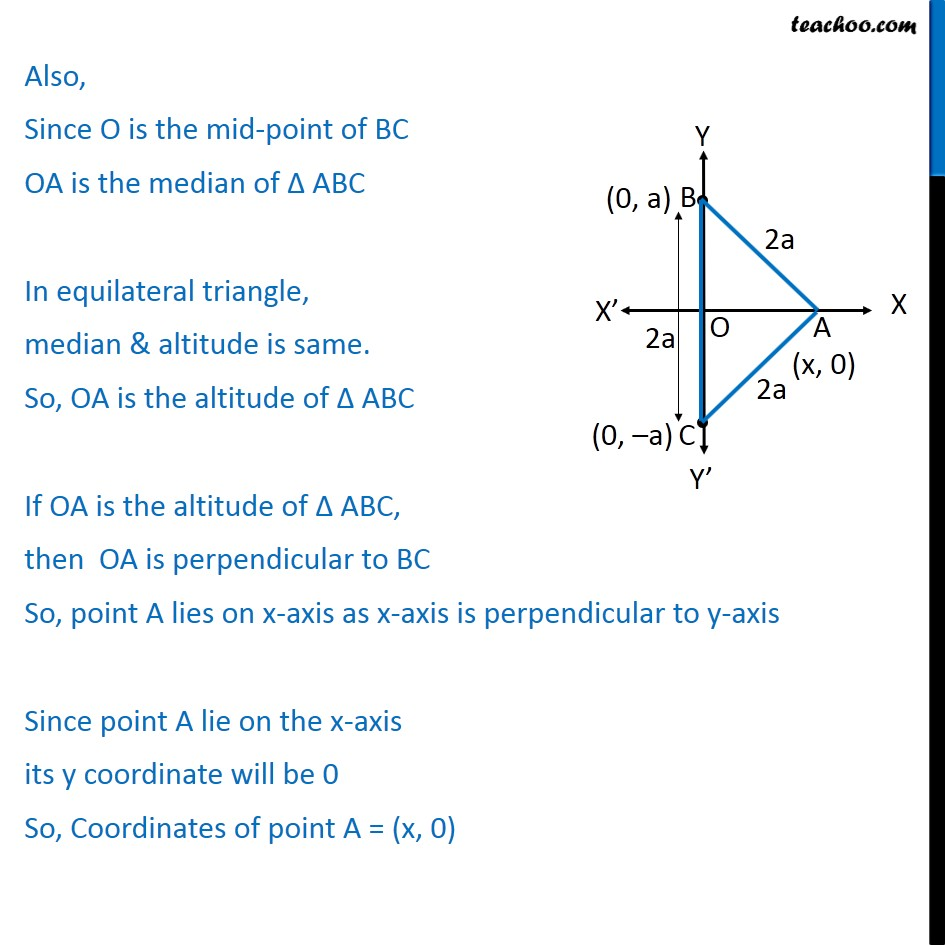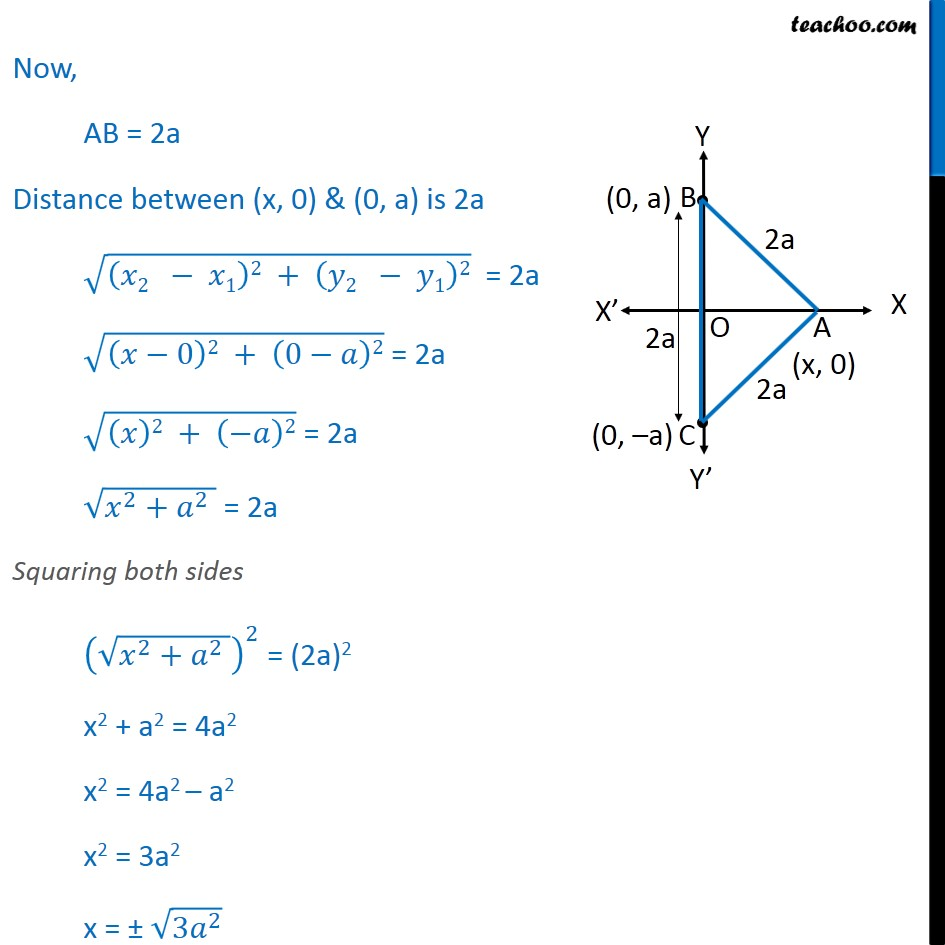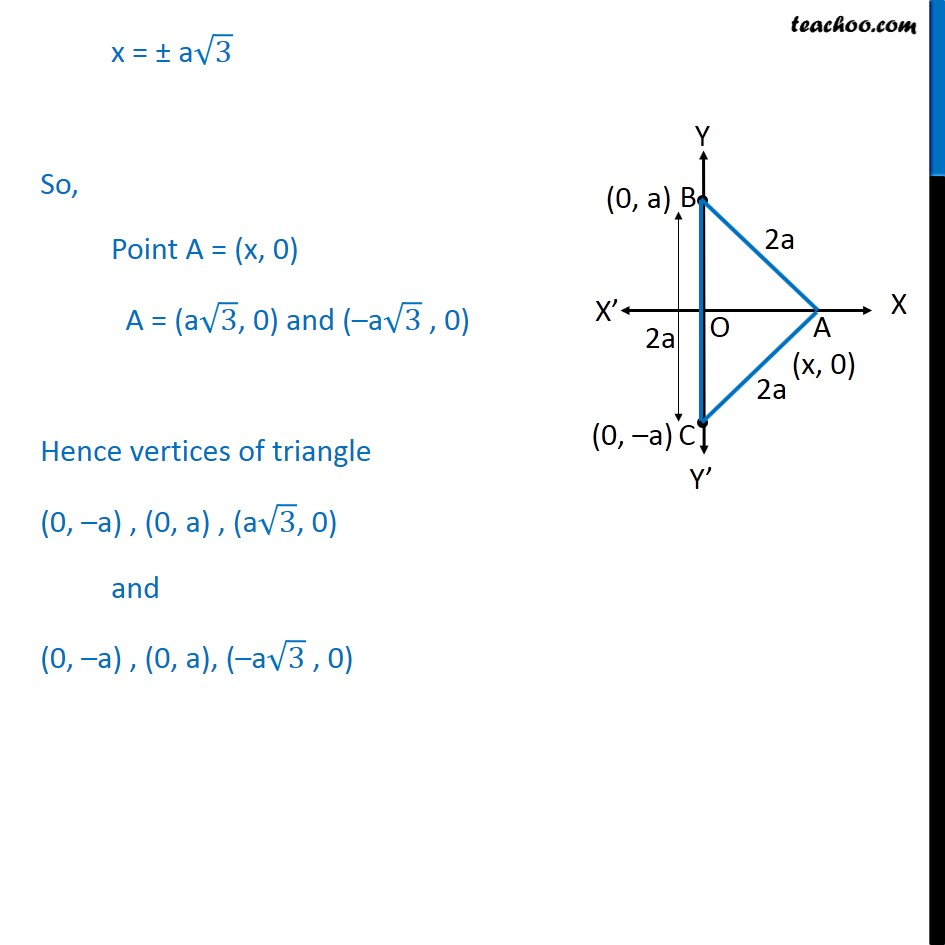Ex 9.1

Chapter 9 Class 11 Straight Lines
Serial order wiseLearn in your speed, with individual attention - Teachoo Maths 1-on-1 Class

### Transcript

Ex10.1, 2 The base of an equilateral triangle with side 2a lies along they y-axis such that the mid point of the base is at the origin. Find vertices of the triangle. Let ABC be an equilateral triangle In equilateral triangle all sides are equal So, AB = BC = AC = 2a Given that the base of equilateral triangle i.e. BC lies along the y-axis such that mid point of BC is O(0, 0) So, OB = OC = a Coordinates of C = (a, 0) & Coordinates of B = ( a, 0) Also, Since O is the mid-point of BC OA is the median of ABC In equilateral triangle, median & altitude is same. So, OA is the altitude of ABC If OA is the altitude of ABC, then OA is perpendicular to BC So, point A lies on y-axis as y-axis is perpendicular to x-axis Since point A lie on the y-axis its x coordinate will be 0 So, Coordinates of point A = (0, y) Now, AB = 2a Distance between (0, y) & ( a, 0) is 2a (( 2 1)2 + ( 2 1)2) = 2a (( 0)2 + (0 )2) = 2a (( )2 + ( )2) = 2a ( ^2+ ^2 ) = 2a Squaring both sides ( ( ^2+ ^2 ))^2 = (2a)2 a2 + y2 = 4a2 y2 = 4a2 a2 y2 = 3a2 y = (3 ^2 ) y = a 3 So, Point A = (0, y) A = (0, a 3) and (0, a 3) Hence vertices of triangle ( 3, 0)(0, -a)(o, -a)& (-a 3, 0)(0, a)&(0, -a) So, Point A = (0, y) A = (0, a 3) and (0, a 3) Hence vertices of triangle ( a, 0) , (a, 0) , (0, a 3) and ( a, 0) , (a, 0), (0, a 3)